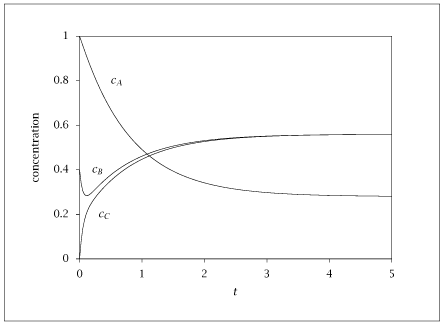## Figure 5.7:

### Full model solution for k_1=1, k_{-1}=0.5, k_2=k_{-2}=10.## Code for Figure 5.7

### main.m

```
1
2
3
4
5
6
7
8
9
10
11
12
13
14
15
16
17
18
19
20
21
22
23
24
25
26
27
28
29
30
31
32
33
34
35
36
37
38
39
40
41
42
43
44
45
46
47
48
49
50
51
52
53
54
55
56
57
58
59
60
61
62
63
64
65
66
67
68
69
70
71
72
73
74% Copyright (C) 2001, James B. Rawlings and John G. Ekerdt
%
% This program is free software; you can redistribute it and/or
% modify it under the terms of the GNU General Public License as
% published by the Free Software Foundation; either version 2, or (at
% your option) any later version.
%
% This program is distributed in the hope that it will be useful, but
% WITHOUT ANY WARRANTY; without even the implied warranty of
% MERCHANTABILITY or FITNESS FOR A PARTICULAR PURPOSE.  See the GNU
% General Public License for more details.
%
% You should have received a copy of the GNU General Public License
% along with this program; see the file COPYING.  If not, write to
% the Free Software Foundation, 59 Temple Place - Suite 330, Boston,
% MA 02111-1307, USA.

k1   = 1;
k_1  = 1/2;
k2   = 10;
k_2  = 10;
c_A0 = 1;
c_B0 = 0.4;
c_C0 = 0;

% nscheme: 0 full model, 1 1st is rls, 2 2nd is rls; 3 qssa on B
nscheme = 0;

if (nscheme == 0)
% IC for full model
x0 = [c_A0; c_B0; c_C0];
elseif (nscheme == 1)
% IC for rx 1 is rls
K2 = k2/k_2;
x0 = [c_A0; 1/(1+K2)*(c_B0+c_C0); K2/(1+K2)*(c_B0+c_C0)];
elseif (nscheme == 2)
% IC for rx 2 is rls
K1 = k1/k_1;
x0 = [1/(1+K1)*(c_A0+c_B0); K1/(1+K1)*(c_A0+c_B0); c_C0];
elseif (nscheme == 3)
% IC for QSSA model
x0 = [c_A0; k1/(k_1+k2)*c_A0 + k_2/(k_1+k2)*c_C0; c_C0];
else
error ('nscheme out of range');
end

p = struct(); % Create structure to pass parameters to ode15s function
p.k1 = k1;
p.k_1 = k_1;
p.k2 = k2;
p.k_2 = k_2;
p.nscheme = nscheme;

tfinal = 5;
ntimes = 200;
tout   = linspace(0, tfinal, ntimes)';

opts = odeset ('AbsTol', sqrt (eps), 'RelTol', sqrt (eps));
[tsolver, x] = ode15s (@(t,x) rhs(t,x,p), tout, x0, opts);

% compute RA RB RC production rates
RA = -k1*x(:,1) + k_1*x(:,2);
RC =  k2*x(:,2) - k_2*x(:,3);
RB = -RA - RC;

table = [tout x RA RB RC];

save -ascii rls_2.dat table;

if (~ strcmp (getenv ('OMIT_PLOTS'), 'true')) % PLOTTING
plot (table(:,1),table(:,2:4));
% TITLE
end % PLOTTING

```

### rhs.m

```
1
2
3
4
5
6
7
8
9
10
11
12
13
14
15
16
17
18
19
20
21
22
23
24
25
26
27
28
29
30
31
32
33
34
35
36
37
38
39
40function retval = rhs(t,x,p)
c_A = x(1);
c_B = x(2);
c_C = x(3);
r1  = p.k1*c_A - p.k_1*c_B;
r2  = p.k2*c_B - p.k_2*c_C;
retval = zeros (3, 1);

if (p.nscheme == 0)
% full model
retval(1) = -r1;
retval(2) = r1 - r2;
retval(3) = r2;
elseif (p.nscheme == 1)
% reaction 1 is rls, reaction 2 at equilibrium
K2 = p.k2/p.k_2;
retval(1) = -r1;
retval(2) = 1/(1+K2)*r1;
retval(3) = K2/(1+K2)*r1;
elseif (p.nscheme == 2)
% reaction 2 is rls, reaction 1 at equilibrium
K1 = p.k1/p.k_1;
retval(1) = -1/(1+K1) * r2;
retval(2) = -K1/(1+K1) * r2;
retval(3) = r2;
elseif (p.nscheme == 3)
% QSSA on B, redefine the c_B concentration under QSSA
c_B = p.k1/(p.k_1 + p.k2)*c_A + p.k_2/(p.k_1 + p.k2)*c_C;
r1 = p.k1*c_A - p.k_1*c_B;
r2 = p.k2*c_B - p.k_2*c_C;
retval(1) = -r1;
%retval(2) = r1 - r2; don't use this one, it is always zero and
%therefore incorrect
retval(2) = p.k1*p.k2*(p.k_2 - p.k1)/...
(p.k_1 + p.k2)^2*c_A + p.k_1*p.k_2*...
(p.k1 - p.k_2)/(p.k_1 + p.k2)^2*c_C;
retval(3) = r2;
else
error ('nscheme out of range');
end

```